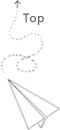# Typical core allocation plan

Some tips about resource allocation scenarios.

When we do the experiments, instead of showing the number of processes, it is also necessary to show how many cores for each proesses. Instead of using the single number, using the A*B form, where A represents the number of processes and B represents how many physical cores is assigned to this process.

Taking the summit node as an exmaple.

Case 1
1 MPI task (process) use 1 core, the program does not support parallel.

Case 2
1 MPI task use one socket (each socket has its memory, NUMA case), the program support the parallel, the number of thread is the number of physical core on its node

Case 3
1 MPI task is running on one node, the program support the parallel, and the number of threads running in parallel is the number of all physical nodes on the machine.

Case 4
Using the GPU as the backend, 1 MPI process can access one GPU, the number of procecss one each node keep same with the number of GPU one each machine. 6 processes in this case. each resource set have 1 GPU and 7 cores.

Case 5

Using the GPU, and each process provides the capability or or associated mechanism to manage multiple GPUs on the current node (such as the pytorch distributed.launch). we need one MPI process on one node.

## 推荐文章